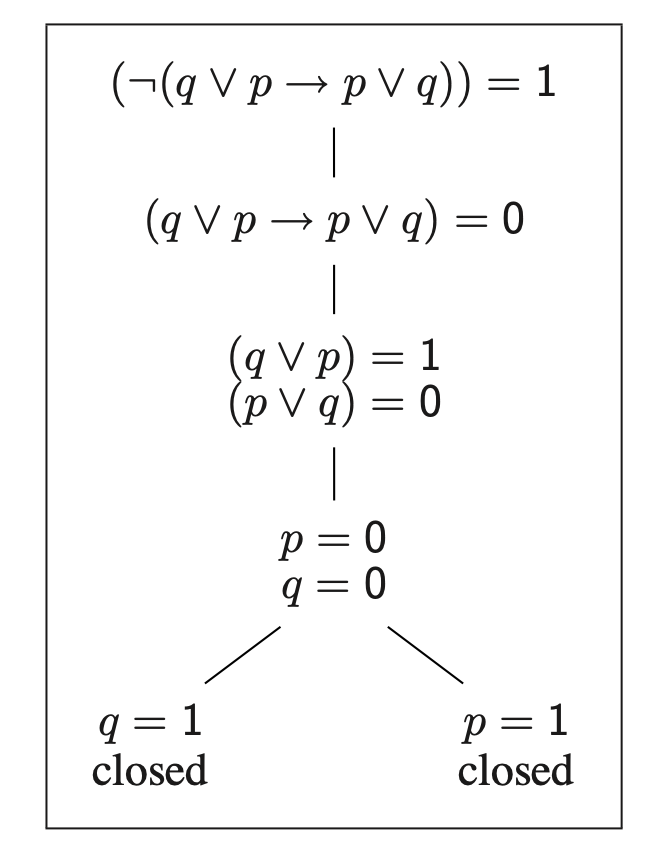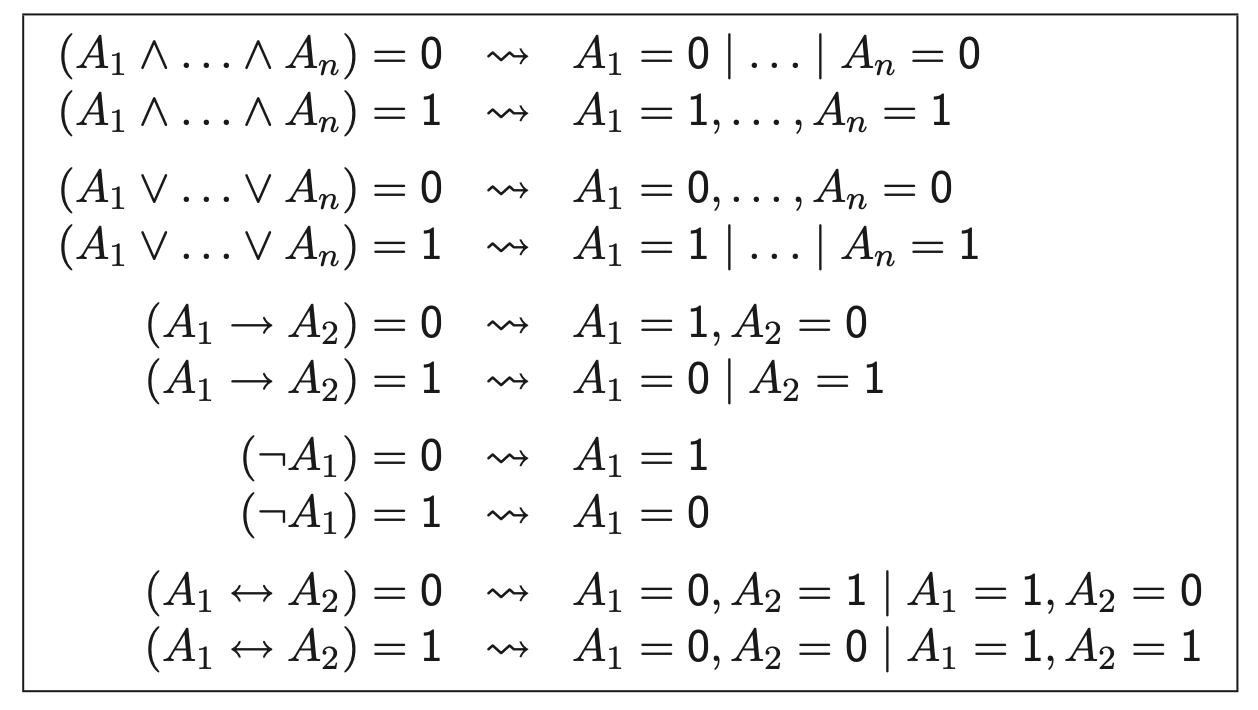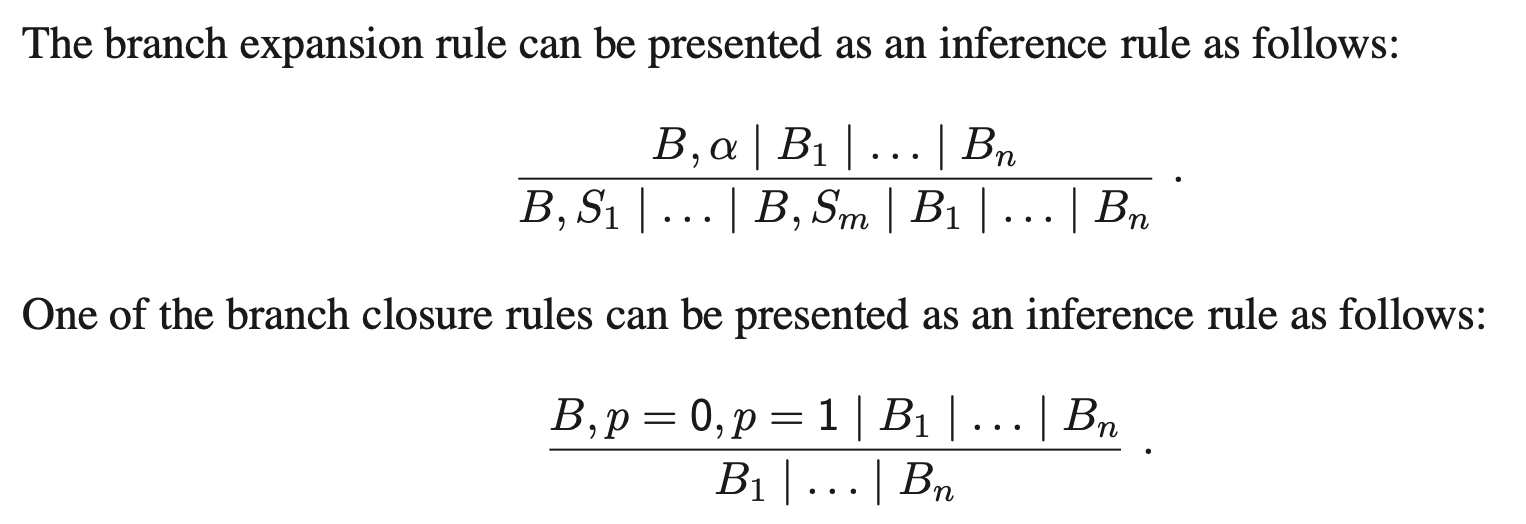# 逻辑学探幽 Part7

## 没有逻辑 只有heip

Posted by R1NG on October 30, 2021 Viewed Times

# 语义树

## 7.1 语义树 (Semantic Tableaux)

$\neg (q \vee p \rightarrow p \vee q)$

$(q \vee p \rightarrow p \vee q)$

$(q \vee p) = 1, ~~ (p \vee q)=0.$

$p=0, ~~ q=0; ~~ q=1 ~\text{or} ~ p=1.$i. 在当前的语义表中选择某个开分支 $B$, 并在该分支上选择一个 有符号公式 $\alpha$, 且该公式需满足 $\alpha$ 不为有符号原子 (也就是说要选择一个尚未被赋值的谓词原子并且同时考虑某个在该分支中包含该原子的子式).

ii. 使用下列的规则扩展语义表:

1. 若 $\alpha$ 形为

$(A_1 \wedge A_2 \wedge \cdots \wedge A_n)=0,$

则将 $\beta$ 作为 $n$ 个子节点 挂在 $B$ 下, 分别为

$A_1=0, A_2=0, \cdots, A_n=0.$
2. 若 $\alpha$ 形为

$(A_1 \wedge A_2 \wedge \cdots \wedge A_n)=1,$

则将 $\beta$ 作为一个 单独子节点 挂在 $B$ 下, 包含公式

$A_1=1, A_2=1, \cdots, A_n=1.$
3. 若 $\alpha$ 形为

$(A_1 \vee A_2 \vee \cdots \vee A_n)=0,$

则将 $\beta$ 作为一个 单独子节点 挂在 $B$ 下, 包含公式

$A_1=0, A_2=0, \cdots, A_n=0.$
4. 若 $\alpha$ 形为

$(A_1 \vee A_2 \vee \cdots \vee A_n)=1,$

则将 $\beta$ 作为 $n$ 个子节点 挂在 $B$ 下, 分别为

$A_1=1, A_2=1, \cdots, A_n=1.$
5. 若 $\alpha$ 形为

$(A_1 \rightarrow A_2)=0,$

则将 $\beta$ 作为一个 单独子节点 挂在 $B$ 下, 包含公式

$A_1=1, A_2=0.$
6. 若 $\alpha$ 形为

$(A_1 \rightarrow A_2)=1,$

则将 $\beta$ 作为 $2$ 个子节点 挂在 $B$ 下, 分别为

$A_1=0, A_2=1.$
7. 若 $\alpha$ 形为

$(\neg A_1)=0,$

则将 $\beta$ 作为一个 单独子节点 挂在 $B$ 下, 包含公式

$A_1=1.$
8. 若 $\alpha$ 形为

$(\neg A_1)=1,$

则将 $\beta$ 作为一个 单独子节点 挂在 $B$ 下, 包含公式

$A_1=0.$
9. 若 $\alpha$ 形为

$(A_1 \leftrightarrow A_2)=0,$

则将 $\beta$ 作为 $2$ 个子节点 挂在 $B$ 下, 分别为

$A_1=0, A_2=1$

$A_1=1, A_2=0.$
10. 若 $\alpha$ 形为

$(A_1 \leftrightarrow A_2)=1,$

$A_1=0, A_2=0$

$A_1=1, A_2=1.$i. 若语义表的某个分支同时包含某个有符号谓词原子 $p$ 的一对值

$p=0, ~~ p=1$

ii. 若语义表的某个分支包含任一赋值

$\top = 0$

$\perp=1$

1. 由于所有分支都闭合, 不再存在任何可能的新决策.
2. 存在一个开分支, 但该分支上的任何有符号公式都已经被选择过, 因而不可再进行任何选择.

$I(p) \overset{\text{def}}{=} \begin{cases} 0~~~ \text{if} ~~ (p=0) \in B \\ 1 ~~~ \text{if} ~~ (p=1) \in B\end{cases}$

## 7.2 可靠性和完备性

1. 有符号谓词公式 $\alpha$ 是可满足的, 当且仅当每一个终止的决策都有一个开分支.
2. 有符号谓词公式 $\alpha$ 是可满足的, 当且仅当每一个终止的决策的分支都是闭的.

1. 若某个语义表的全部分支都是闭分支, 则 $\gamma$ 为不可满足的.
2. 若在决策过程终止后语义表中仍存在一开分支, 则我们可从这一分支中提取出 $\gamma$ 的一个模型.

1. 一对原子 $p$ 的相反赋值 $p=1, ~ p=0$
2. 至少包含 $\top = 0$ 或 $\perp = 1$ 其中一个 则称该集合是 平凡地不可接受的.

1. 若 $S$ 包含一个有符号公式 $(A_1 \wedge A_2 \wedge \cdots \wedge A_n)=0$, 则 $S$ 同时至少包含有符号公式 $A_1=0, A_2=0, \cdots, A_n=0$ 中的至少一个.

2. 若 $S$ 包含一个有符号公式 $(A_1 \wedge A_2 \wedge \cdots \wedge A_n)=1$, 则 $S$ 同时至少包含有符号公式 $A_1=1, A_2=1, \cdots, A_n=1$ 中的至少一个.

3. 若 $S$ 包含一个有符号公式 $(A_1 \vee A_2 \vee \cdots \vee A_n)=0$, 则 $S$ 同时包含有符号公式 $A_1=0, A_2=0, \cdots, A_n=0$.

4. 若 $S$ 包含一个有符号公式 $(A_1 \vee A_2 \vee \cdots \vee A_n)=1$, 则 $S$ 同时包含有符号公式 $A_1=1, A_2=1, \cdots, A_n=1$.

5. 若 $S$ 包含一个有符号公式 $(A_! \rightarrow A_2)=0$, 则 $S$ 同时包含有符号公式 $A_1=0, A_2=0$.

6. 若 $S$ 包含一个有符号公式 $(A_! \rightarrow A_2)=0$, 则 $S$ 或包含有符号公式 $A_1=0$, 或包含 $A_2=1$.

7. 若 $S$ 包含一个有符号公式 $\neg A_1 = 0$, 则 $S$ 同时包含 $A_1 = 1$.

8. 若 $S$ 包含一个有符号公式 $\neg A_1 = 1$, 则 $S$ 同时包含 $A_1 = 0$.

9. 若 $S$ 包含一个有符号公式 $A_1 \leftrightarrow A_2=0$, 则 $S$ 同时包含 $A_1 = 0, ~ A_2=1$, 或 $A_1 = 1, ~ A_2=0$.

10. 若 $S$ 包含一个有符号公式 $A_1 \leftrightarrow A_2=1$, 则 $S$ 同时包含 $A_1 = 0, ~ A_2=0$, 或 $A_1 = 1, ~ A_2=1$.

$I(p) \overset{\text{def}}{=} \begin{cases} 0~~~ \text{if} ~~ (p=0) \in B \\ 1 ~~~ \text{if} ~~ (p=1) \in B\end{cases}$

1. 谓词公式 $F$ 是可满足的, 当且仅当对于任意有符号谓词公式 $F=1$ 的终止的语义表都具一个开分支.
2. 谓词公式 $F$ 是有效 (valid)的, 当且仅当对于任意有符号谓词公式 $F=0$ 的终止的语义表的分支都是闭分支.
3. 谓词公式 $F_1, F_2$ 为等价的, 当且仅当任意对有符号谓词公式 $(F_1 \leftrightarrow F_2) = 0$ 的终止的语义表的分支都是闭分支.

## 7.3 语义树的另一种解释法

$\{B_1, \cdots, B_n\}$

$B_1 \vert \cdots \vert B_n.$

$n=0$ 时, 称其为 空语义表, 记为 $\boxplus$. 称解释 $I$ 为 语义表 $T$ 的一个模型, 若 $I$ 为该表中 至少一个分支 的模型.

1. 空语义表不存在任何模型.
2. 若某个语义表只包含一条分支, 则该表示可满足的, 当且仅当它所包含的唯一分支是可满足的.

1. 分支扩充规则.
考虑在第一节中介绍的 规则 7.1.1. 对于其中任意一条规则, 若它形如

$\alpha \rightsquigarrow S_1 \vert \dots S_m$

且语义表 $T$ 包含分支 $B$, 分支 $B$ 中的谓词公式 $\alpha$ 包含 $\alpha$, 则将 $T$ 中该分支 $B$ 替代为 $m$ 个新分支

$B \cup S_1, ~ B \cup S_2, \cdots, B\cup S_m.$
1. 分支闭合规则
若某分支包含有符号谓词公式

$\top = 0, ~ \perp = 1$

中的至少一个或一对互相矛盾的谓词原子取值

$p=0, ~~ p=1$

则可从语义表中删除这条分支.1. 该表中只包含有符号原子和有符号公式 $\top = 0, ~ \perp = 1$.
2. 表中不存在互相矛盾的谓词原子取值.
3. 表中的每一条分支都是可满足的.
4. 该表为可满足的, 当且仅当该表非空.

1. 存在一个从 $\gamma$ 到 $T$ 的推导 (Derivation).
2. 任何推断规则都不可应用于 $T$ 上.